# Lions Weekly: April 6, 2022# A Note From Mr. Sandefur

Lion Family,

Good afternoon! I wanted to update you on a conversation I recently had with a new friend of mine, Ira, in Ukraine. I had the humbling opportunity, through TKA parent Greg Gibson, to connect with Ira, who serves as the Principal of a Christian school in Western Ukraine. I was able to learn of ways that our TKA family can intercede on their behalf in prayer, as well as efforts that can sustain their ability to teach generations of Ukrainians from a biblically-based worldview in the future. We will be sending a comprehensive prayer email to all stakeholders in the near future that details how our TKA family can be praying for our brothers and sisters in Ukraine as they seek to continue educating their children while serving refugees and those impacted by war.

On that note, I would like to ask that you join me in praying specifically for Mrs. Madison Guillion and Mrs. Iyrna Brooks, who are traveling to Romania this week to serve Ukrainian refugees. These incredible teachers are giving of their time and energy to serve those in need during this difficult time. I am very proud of our school body as they seek to make a difference in our community (serving those impacted by the wildfires) and throughout our world. You have heard me say many times that we desire to develop servant leaders who will change the world for Christ, and it is humbling to see evidence of that everywhere we look throughout our school community.

In Christ Alone,

Jeremy Sandefur
President

 table div table+table+table+table div table{width:100%;padding:0}table div table+table+table+table div table img{width:96.23%;padding:0;float:none}table div table+table+table+table div table td{width:100%;padding:0 1.88% 18px}/* styles */Mrs. Hatmaker and Mr. Fuller will host current 8th Graders and their families in Woody Auditorium at 3:15 this Thursday to discuss what to expect as they move into High School. The discussion will include graduation requirements, GPA, and much more!

 table div table+table+table+table+table+table div table{width:100%;padding:0}table div table+table+table+table+table+table div table img{width:96.23%;padding:0;float:none}table div table+table+table+table+table+table div table td{width:100%;padding:0 1.88% 18px}/* styles */# Mother vs. Son Kickball Tournament

Who: Prek3- 5th grade students and their mom (grandmother, aunt, or important female figure)

When: Saturday, April 30th
Lunch is from 11:00a.m. to 1:00 p.m.
Games begin at 1:15

Where: Dr. Carroll Reece Reagan Pavilion

Cost: \$5.00 per person eating

Any questions regarding this event, please email Ashley Dawson at ashleydawson82@gmail.com

For those planning on attending the event, please fill out this form and return it with the payment by Friday, April 15.

 table div table+table+table+table+table+table+table+table div table{width:100%;padding:0}table div table+table+table+table+table+table+table+table div table img{width:96.23%;padding:0;float:none}table div table+table+table+table+table+table+table+table div table td{width:100%;padding:0 1.88% 18px}/* styles */# Prom Information

DATE: April 9, 2022
PLACE: Capitol Theatre, Maryville, TN
TIME: 6:30 pm - 10:30 pm

TICKETS: \$55 per person

Purchase tickets here

Desserts/Light Refreshments will be served.

ALL outside guests must be approved via the attached form.

DRESS CODE REQUIREMENTS:
Girls - Prom and homecoming dresses must be viewed on the individual in advance for suitable fitting - no exceptions. Skirts of the dresses may not be sheer (see through) material. “Dollar Bill” test will be used for the length. Length should be no shorter than width of dollar bill above knee (approximately two inches). Neckline and backline must be modest (backline must be above the natural waistline). Spaghetti straps and strapless styles are acceptable, if modest. The midriff should always remain covered. No open sides or sheer panels. No immodest tight dresses are allowed. Viewing dates will be provided for both homecoming and prom.

Boys - Suit & Tie or Tuxedo, Dress Shoes

Prom Dress Checks will be:
via email to lwallace@thekingsacademy.net and kpolk@thekingsacademy.net - send pics of front and back full length of dress in good lighting.

## Contact Jenny Cook via text 865-387-3468 with any prom related questions.

 table div table+table+table+table+table+table+table+table+table+table div table{width:100%;padding:0}table div table+table+table+table+table+table+table+table+table+table div table img{width:96.23%;padding:0;float:none}table div table+table+table+table+table+table+table+table+table+table div table td{width:100%;padding:0 1.88% 18px}/* styles */# 2023 Europe Trip Interest Meeting

Are you a high school student (in grades 9 - 12 for the 2022-23 school year), or do you have a high school student who would be interested in attending the 2023 Europe Trip to France, Germany, and the Alps?

 table div table+table+table+table+table+table+table+table+table+table+table+table+table div table{width:100%;padding:0}table div table+table+table+table+table+table+table+table+table+table+table+table+table div table img{width:96.23%;padding:0;float:none}table div table+table+table+table+table+table+table+table+table+table+table+table+table div table td{width:100%;padding:0 1.88% 18px}/* styles */TKA's middle and high school art department will be showcasing their amazing work on their new instagram site. Click here to follow them and see the great things happening in the art department!

 table div table+table+table+table+table+table+table+table+table+table+table+table+table+table+table div table{width:100%;padding:0}table div table+table+table+table+table+table+table+table+table+table+table+table+table+table+table div table img{width:96.23%;padding:0;float:none}table div table+table+table+table+table+table+table+table+table+table+table+table+table+table+table div table td{width:100%;padding:0 1.88% 18px}/* styles */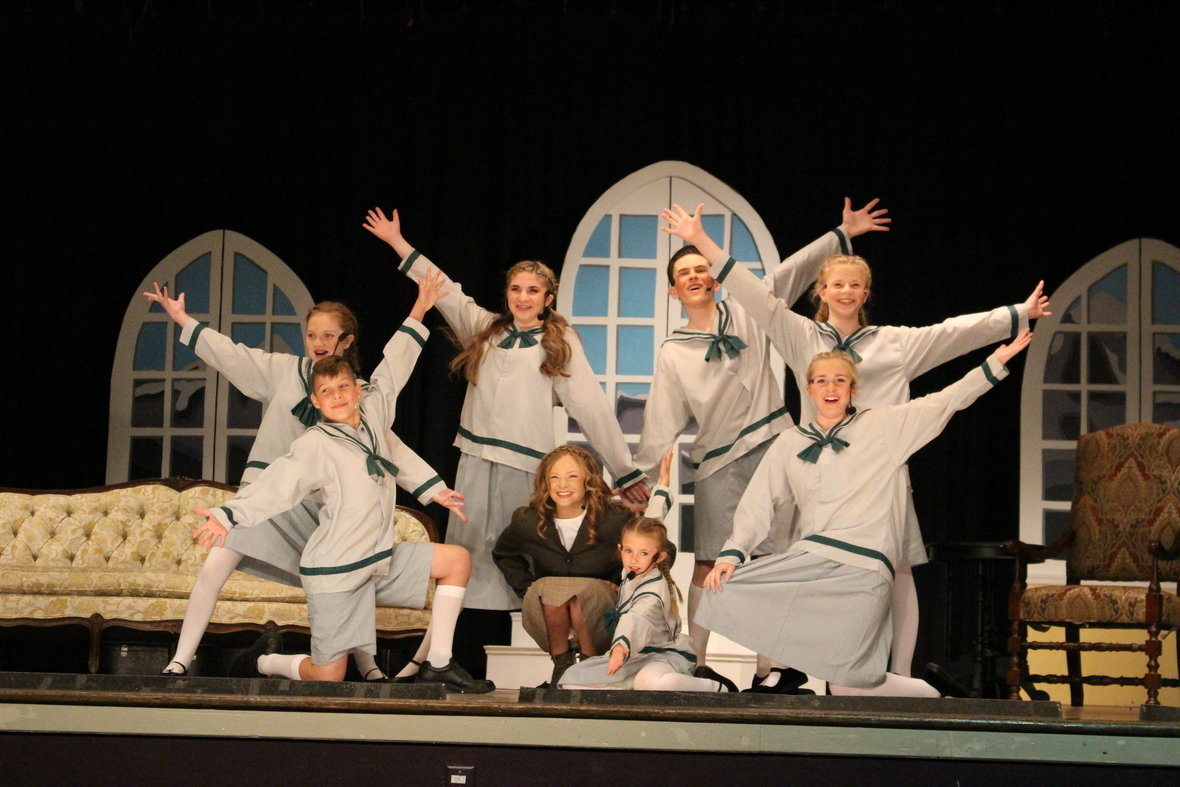# TKA's Drama and Music Departments

Last weekend, the drama and music departments presented four performances of The Sound of Music! The cast and crew did a phenomenal job! Take a look at some of the pictures from the show.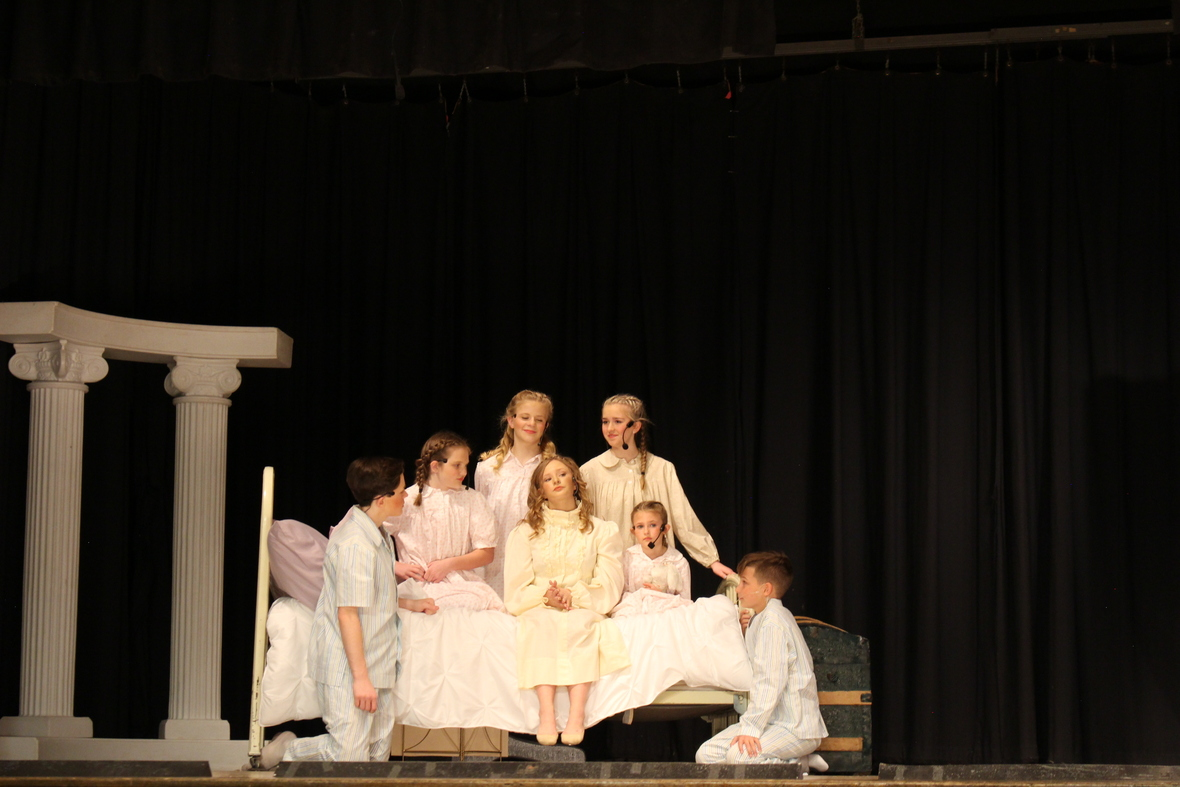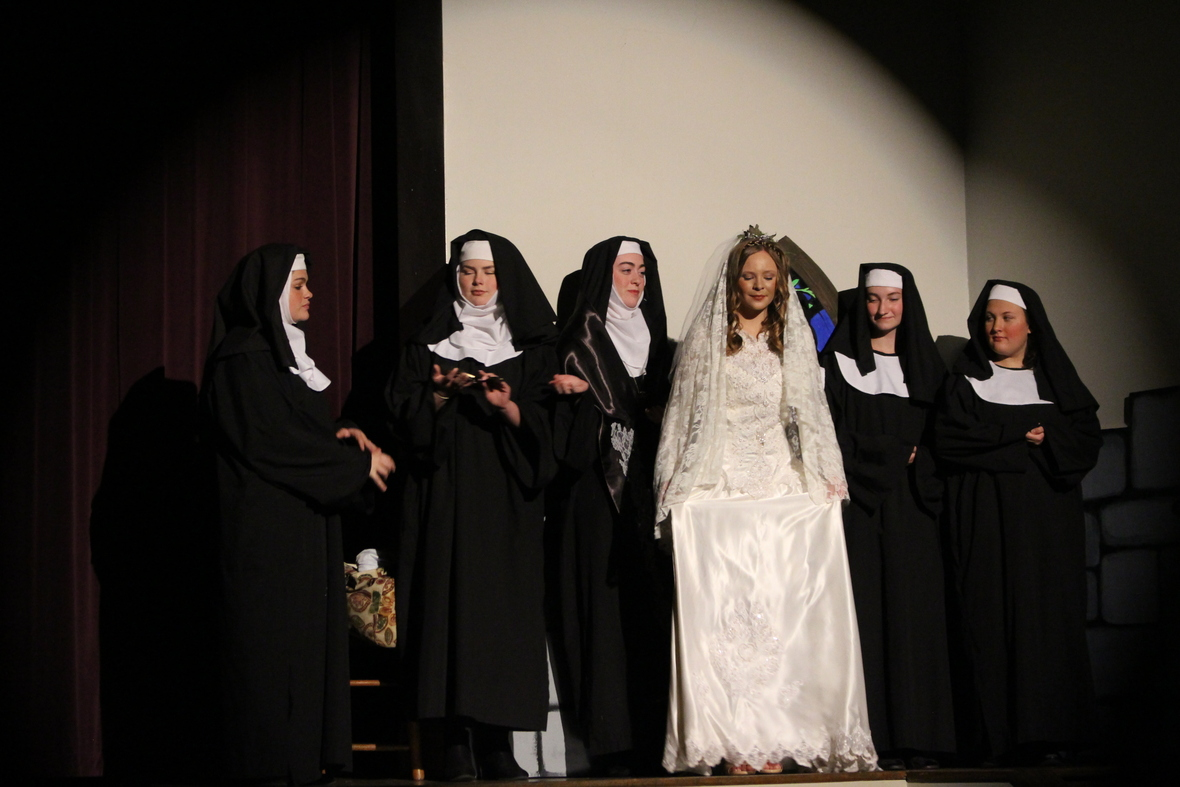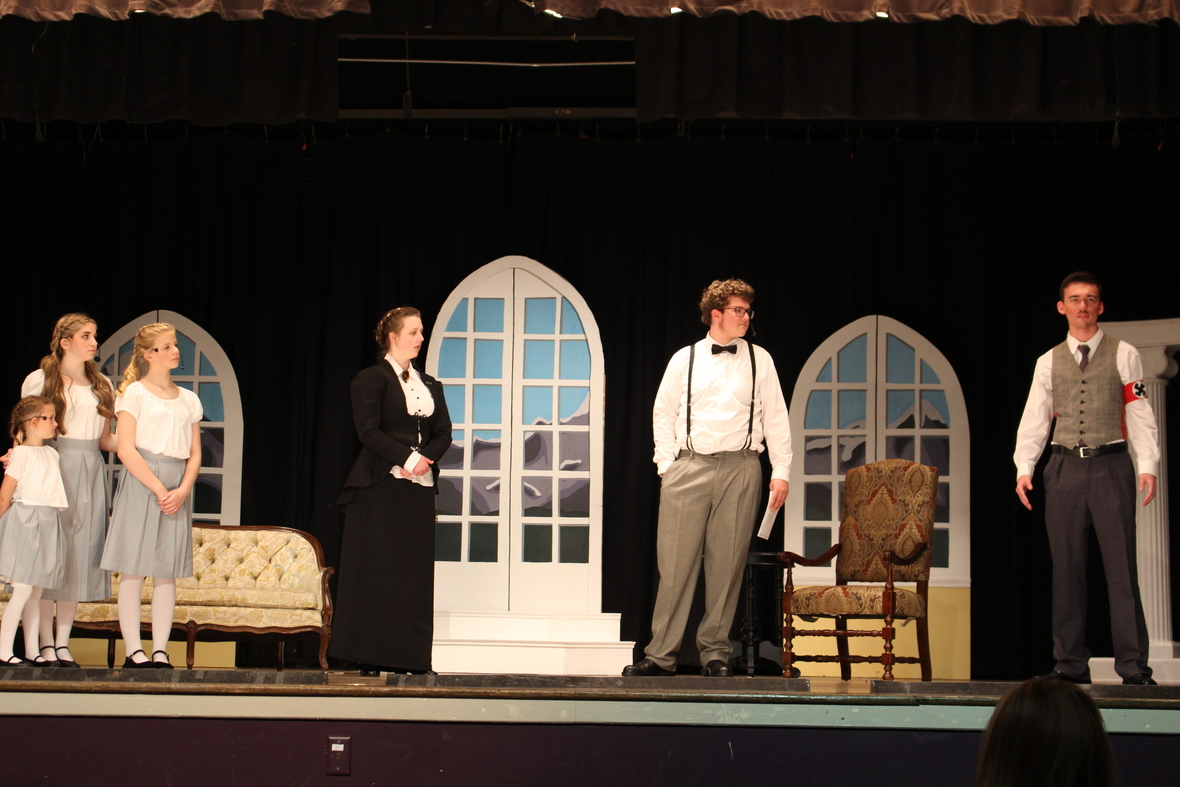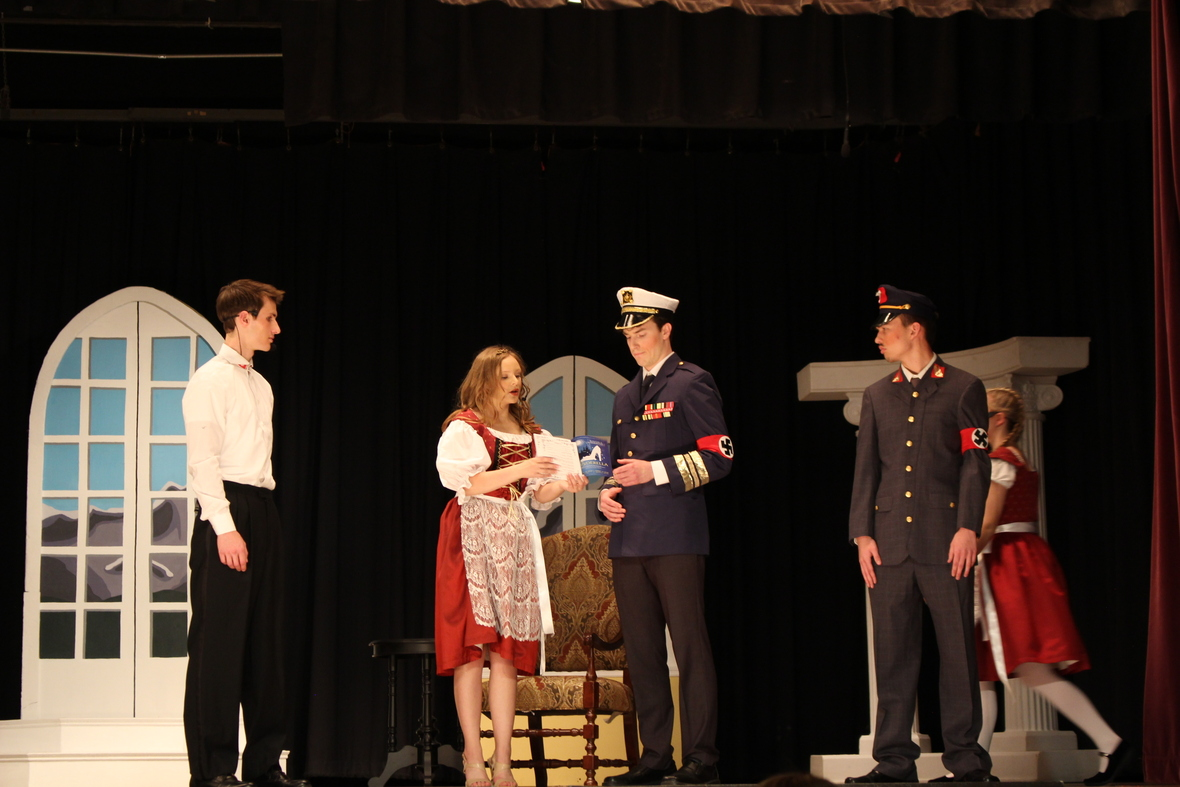table div table+table+table+table+table+table+table+table+table+table+table+table+table+table+table+table+table+table+table+table div table{width:100%;padding:0}table div table+table+table+table+table+table+table+table+table+table+table+table+table+table+table+table+table+table+table+table div table img{width:96.23%;padding:0;float:none}table div table+table+table+table+table+table+table+table+table+table+table+table+table+table+table+table+table+table+table+table div table td{width:100%;padding:0 1.88% 18px}/* styles */# End of the Year Events

As the end of the year quickly approaches, we want to be sure and communicate any calendar events as soon as we are able. Below are upcoming events for the Month of May we would like for you to mark on your calendar.
Tuesday, May 3
- Middle and High School Band Concert at 6:30 p.m. in Woody Auditorium
Wednesday, May 4
- Jr. Beta \$1 Star Wars dress up day to benefit Isaiah 117 House
Thursday, May 5
- Middle and High School Choir Concert at 6:30 p.m. in Woody Auditorium
Friday, May 6:
- Elementary Field Day from 8:30 a.m. to 2:00 p.m. (Huskey Field)
Saturday, May 7:
- PTF Family Fun Fair from 10:00 a.m. to 2:00 p.m.
Thursday, May 12:
- Elementary Spring Concert at 6:30 p.m. in Woody Auditorium
Friday, May 13:
- Senior Breakfast at 8:00 a.m.
- Legacy Walk at 9:00 a.m.
- Big/Little Buddy End of the Year Party at 10:30 a.m.
Sunday, May 15
- Baccalaureate Service at 3:00 p.m. at Seymour First Baptist
Tuesday, May 17
Thursday, May 19
- PTF Meeting at 3:15 in Brandes
Friday, May 20:
- High School Awards Day at 10:00 a.m. in Tibbetts
- Senior Graduation at 7:00 p.m. in Tibbetts
Monday, May 23:
- PreK4 Graduation at 6:00 p.m. in Woody Auditorium
Tuesday, May 24:
- HS Exams
- Kindergarten Graduation at 6:00 p.m. in Woody Auditorium
Wednesday, May 25
- HS Exams
Thursday, May 26:
- HS Exams
- 5th Grade promotion at 8:15 a.m. to 9:15 a.m. in Woody Auditorium
- Elementary classroom end of the year programs
Friday, May 27:
- Make-up HS Exams
- Middle School Awards at 9:00 a.m. in Woody Auditorium
- 8th Grade Promotion at 10:00 a.m. in Woody Auditorium
- Half-day dismissal at 11:30 a.m. No aftercare will be provided.

 table div table+table+table+table+table+table+table+table+table+table+table+table+table+table+table+table+table+table+table+table+table+table div table{width:100%;padding:0}table div table+table+table+table+table+table+table+table+table+table+table+table+table+table+table+table+table+table+table+table+table+table div table img{width:96.23%;padding:0;float:none}table div table+table+table+table+table+table+table+table+table+table+table+table+table+table+table+table+table+table+table+table+table+table div table td{width:100%;padding:0 1.88% 18px}/* styles */Calling all upcoming Middle and High school Lions interested in cheering for the 2022-2023 school year! If you or someone you know is interested in cheering for TKA during the 2022-2023 school year, this information is for you! if you were unable to attend these meetings but are interested in being a middle or high school cheerleader, please contact Coach Natoisha Hager at nhager@thekingsacademy.net.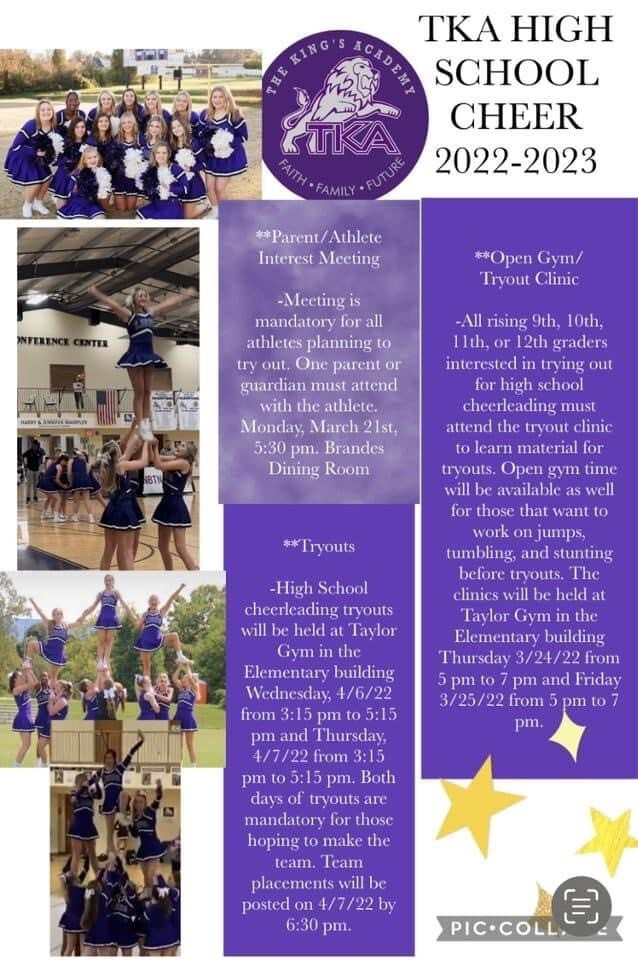table div table+table+table+table+table+table+table+table+table+table+table+table+table+table+table+table+table+table+table+table+table+table+table+table+table div table{width:100%;padding:0}table div table+table+table+table+table+table+table+table+table+table+table+table+table+table+table+table+table+table+table+table+table+table+table+table+table div table img{width:96.23%;padding:0;float:none}table div table+table+table+table+table+table+table+table+table+table+table+table+table+table+table+table+table+table+table+table+table+table+table+table+table div table td{width:100%;padding:0 1.88% 18px}/* styles */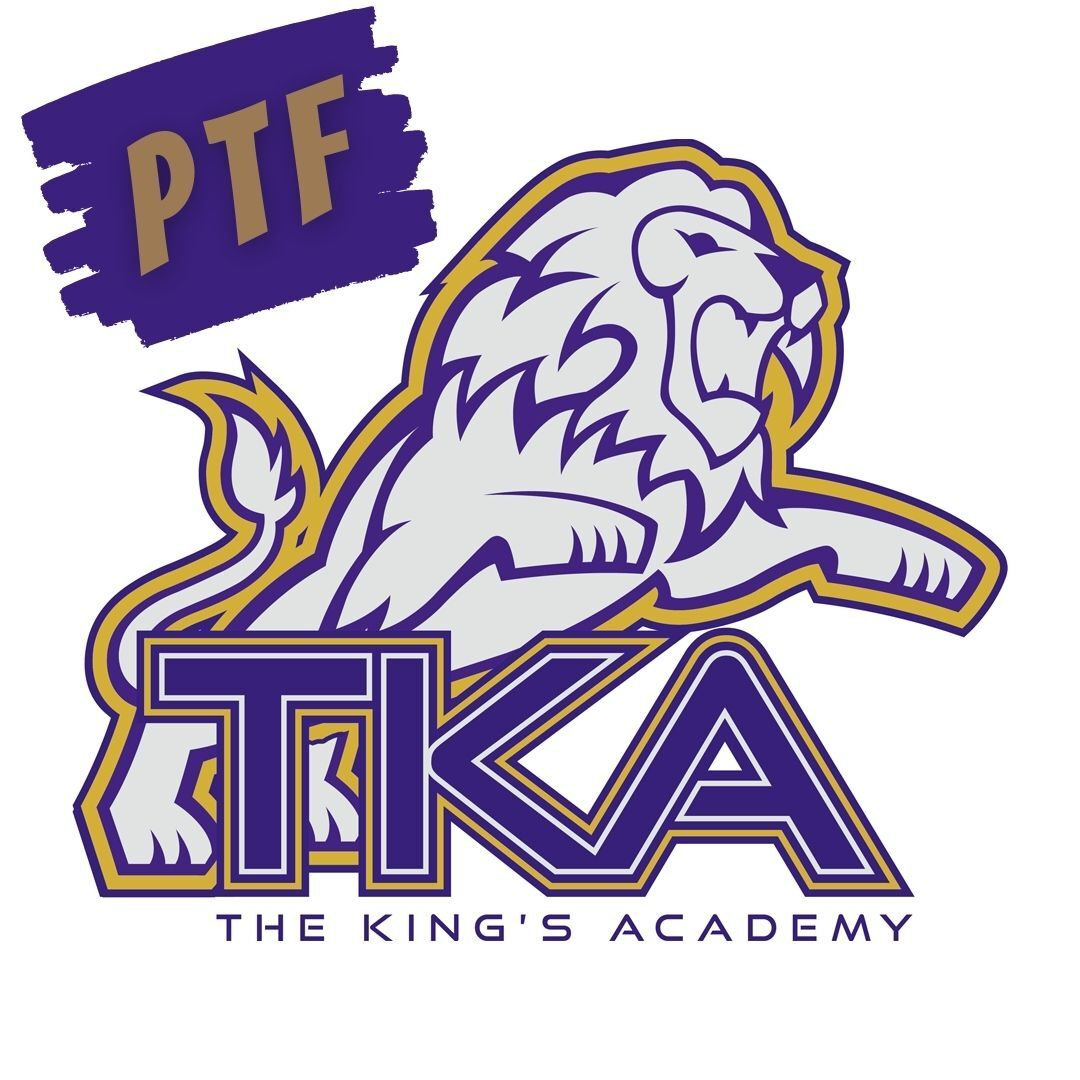# Save these Dates!

Below are the upcoming PTF meetings for the remainder of the year from 3:15 - 4:15 in Brandes:

April 28
May 19

PTF will host the Family Fun Fair on Saturday, May 7, from 10:00 a.m. to 2:00 p.m. This event will take place rain or shine. Join us for a day of fellowship and fun for all ages including inflatables, cakewalk, vendors, food truck and so much more!

 table div table+table+table+table+table+table+table+table+table+table+table+table+table+table+table+table+table+table+table+table+table+table+table+table+table+table+table div table{width:100%;padding:0}table div table+table+table+table+table+table+table+table+table+table+table+table+table+table+table+table+table+table+table+table+table+table+table+table+table+table+table div table img{width:96.23%;padding:0;float:none}table div table+table+table+table+table+table+table+table+table+table+table+table+table+table+table+table+table+table+table+table+table+table+table+table+table+table+table div table td{width:100%;padding:0 1.88% 18px}/* styles */# Elementary Summer Tutoring

Thank you to all of the elementary parents that reached out to Ms. Addison regarding summer tutoring. At this time, all of her tutoring spots have been filled. If you signed up, Ms. Addison will be reaching out to you directly regarding your Lion's summer tutoring.

 table div table+table+table+table+table+table+table+table+table+table+table+table+table+table+table+table+table+table+table+table+table+table+table+table+table+table+table+table+table div table{width:100%;padding:0}table div table+table+table+table+table+table+table+table+table+table+table+table+table+table+table+table+table+table+table+table+table+table+table+table+table+table+table+table+table div table img{width:96.23%;padding:0;float:none}table div table+table+table+table+table+table+table+table+table+table+table+table+table+table+table+table+table+table+table+table+table+table+table+table+table+table+table+table+table div table td{width:100%;padding:0 1.88% 18px}/* styles */# 27th Annual Golf Tournament

Join us at Egwani Farms on June 13, for our 27th Annual Golf Tournament to benefit TKA's football team!

Gather your company, friends or family and purchase one of the following sponsorships:
- Purple Sponsor includes a 4-person team and a hole sponsorship for \$500
- Silver Sponsor includes an individual player and a hole sponsorship for \$200
- Individual players can participate for \$100

 table div table+table+table+table+table+table+table+table+table+table+table+table+table+table+table+table+table+table+table+table+table+table+table+table+table+table+table+table+table+table+table div table{width:100%;padding:0}table div table+table+table+table+table+table+table+table+table+table+table+table+table+table+table+table+table+table+table+table+table+table+table+table+table+table+table+table+table+table+table div table img{width:96.23%;padding:0;float:none}table div table+table+table+table+table+table+table+table+table+table+table+table+table+table+table+table+table+table+table+table+table+table+table+table+table+table+table+table+table+table+table div table td{width:100%;padding:0 1.88% 18px}/* styles */# Upcoming Sports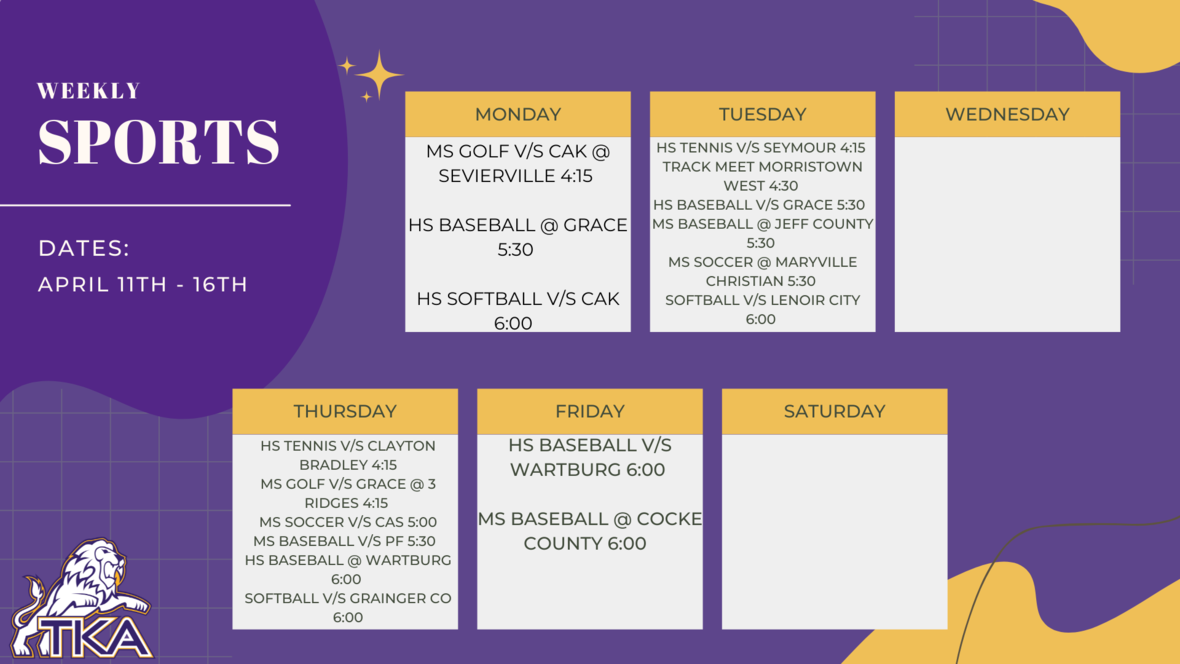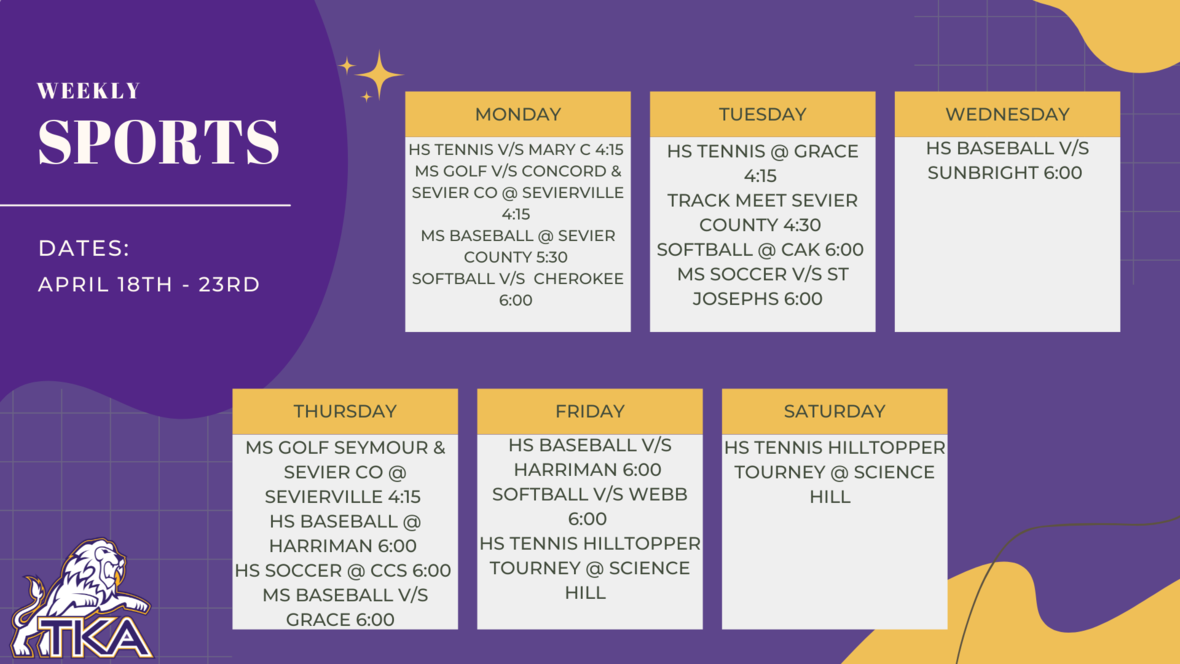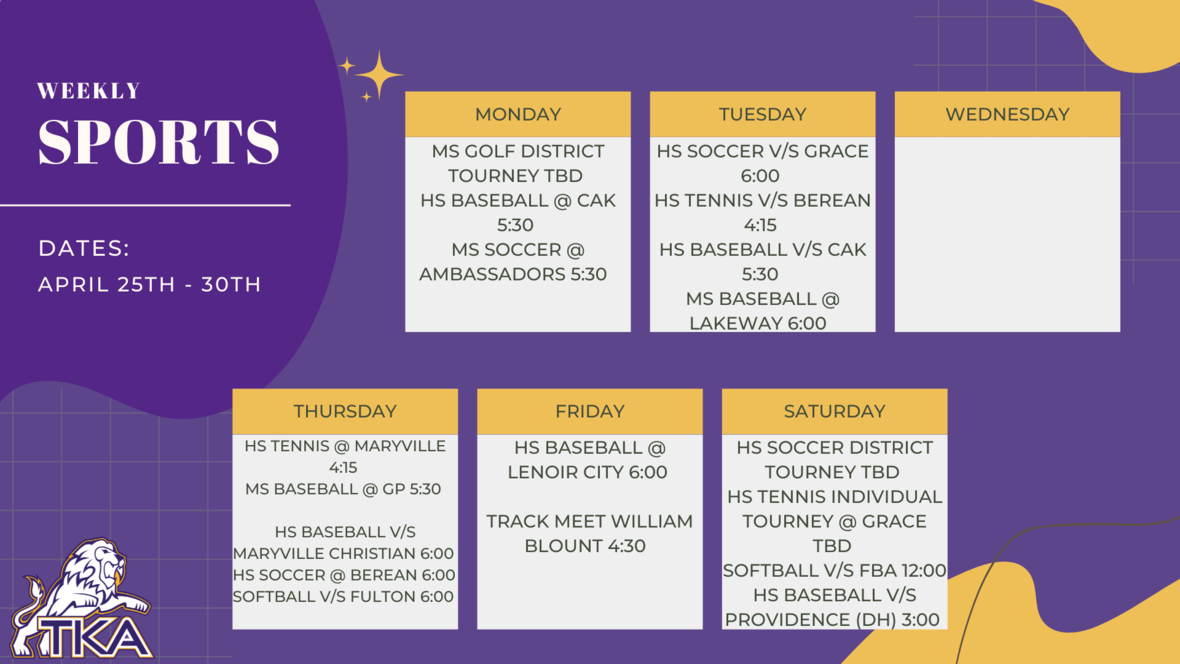table div table+table+table+table+table+table+table+table+table+table+table+table+table+table+table+table+table+table+table+table+table+table+table+table+table+table+table+table+table+table+table+table+table+table+table+table+table div table{width:100%;padding:0}table div table+table+table+table+table+table+table+table+table+table+table+table+table+table+table+table+table+table+table+table+table+table+table+table+table+table+table+table+table+table+table+table+table+table+table+table+table div table img{width:96.23%;padding:0;float:none}table div table+table+table+table+table+table+table+table+table+table+table+table+table+table+table+table+table+table+table+table+table+table+table+table+table+table+table+table+table+table+table+table+table+table+table+table+table div table td{width:100%;padding:0 1.88% 18px}/* styles */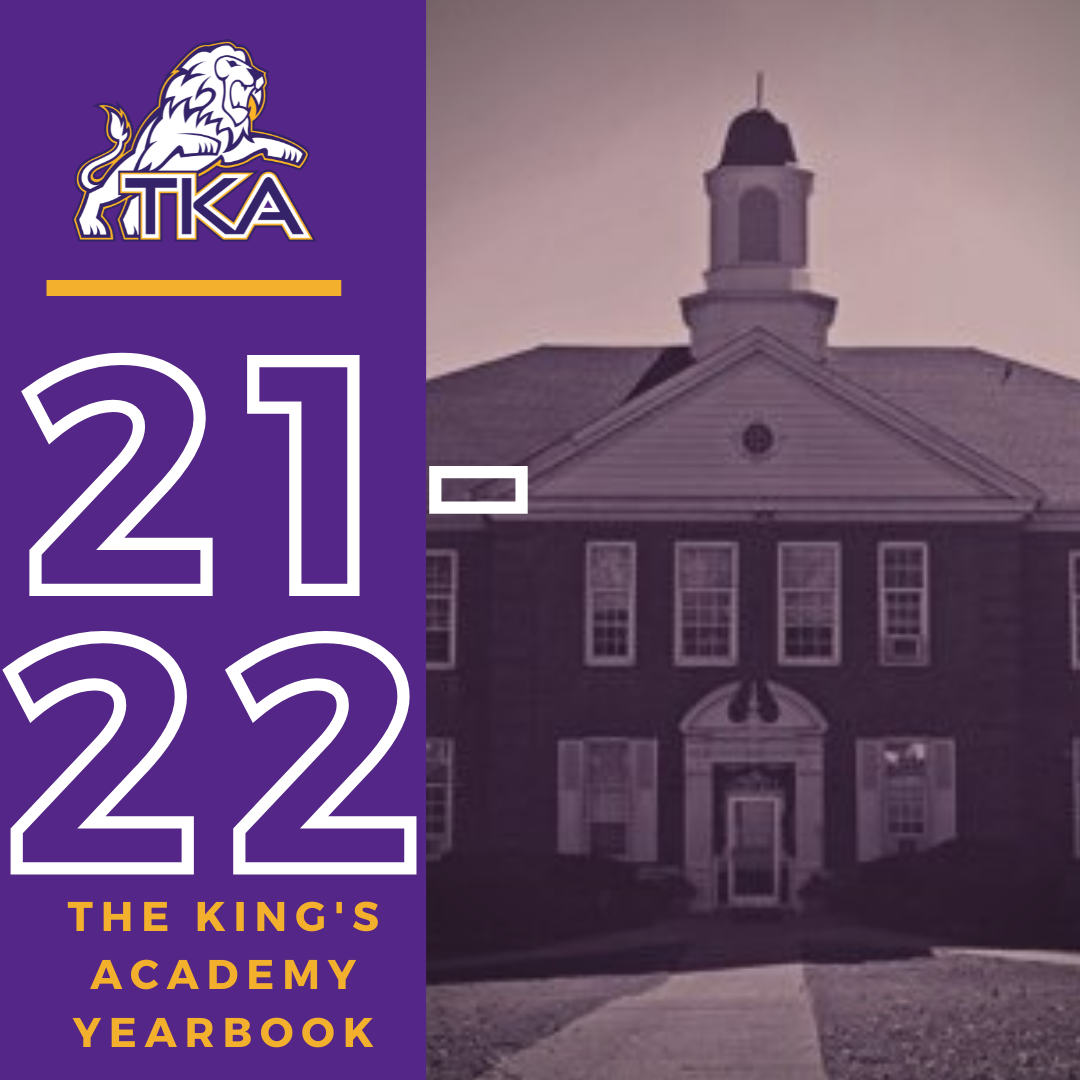# 2021-2022 Yearbook

The price for the 2021-2022 TKA yearbook is \$55. All grades, K3-12 will have the same yearbook. The slight price increase is because we are only going to have one larger all-school book.

All yearbook orders can be placed online--here is the link.Do you have a class of 2022 senior in your household?

Don't miss out on the opportunity to purchase a Senior Tribute for the 2021-2022 yearbook!

Senior Tributes are available for purchase at the bottom of the link listed here.For any questions regarding yearbooks, email Mr. Josh Smith at jsmith@thekingsacademy.net.

 table div table+table+table+table+table+table+table+table+table+table+table+table+table+table+table+table+table+table+table+table+table+table+table+table+table+table+table+table+table+table+table+table+table+table+table+table+table+table+table+table+table div table{width:100%;padding:0}table div table+table+table+table+table+table+table+table+table+table+table+table+table+table+table+table+table+table+table+table+table+table+table+table+table+table+table+table+table+table+table+table+table+table+table+table+table+table+table+table+table div table img{width:96.23%;padding:0;float:none}table div table+table+table+table+table+table+table+table+table+table+table+table+table+table+table+table+table+table+table+table+table+table+table+table+table+table+table+table+table+table+table+table+table+table+table+table+table+table+table+table+table div table td{width:100%;padding:0 1.88% 18px}/* styles */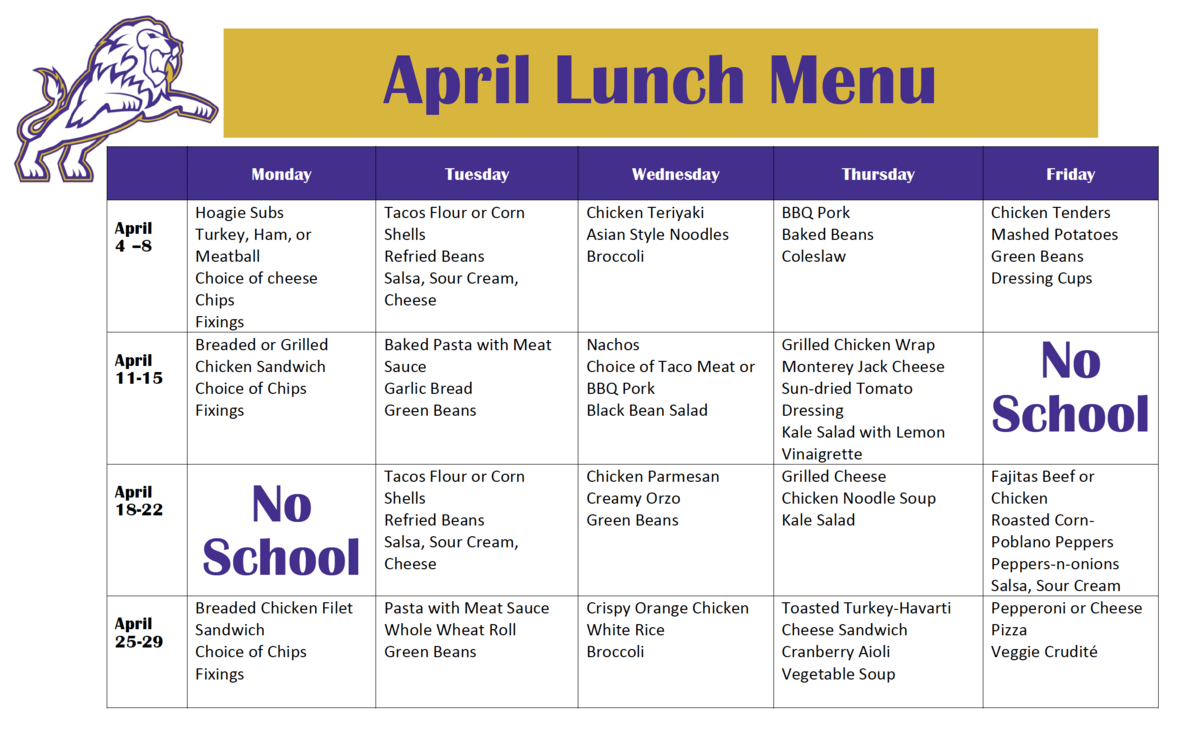table div table+table+table+table+table+table+table+table+table+table+table+table+table+table+table+table+table+table+table+table+table+table+table+table+table+table+table+table+table+table+table+table+table+table+table+table+table+table+table+table+table+table+table div table{width:100%;padding:0}table div table+table+table+table+table+table+table+table+table+table+table+table+table+table+table+table+table+table+table+table+table+table+table+table+table+table+table+table+table+table+table+table+table+table+table+table+table+table+table+table+table+table+table div table img{width:96.23%;padding:0;float:none}table div table+table+table+table+table+table+table+table+table+table+table+table+table+table+table+table+table+table+table+table+table+table+table+table+table+table+table+table+table+table+table+table+table+table+table+table+table+table+table+table+table+table+table div table td{width:100%;padding:0 1.88% 18px}/* styles *//* styles */
 table div table+table+table+table+table+table+table+table+table+table+table+table+table+table+table+table+table+table+table+table+table+table+table+table+table+table+table+table+table+table+table+table+table+table+table+table+table+table+table+table+table+table+table+table+table+table div table{width:100%;padding:0}table div table+table+table+table+table+table+table+table+table+table+table+table+table+table+table+table+table+table+table+table+table+table+table+table+table+table+table+table+table+table+table+table+table+table+table+table+table+table+table+table+table+table+table+table+table+table div table img{width:96.23%;padding:0;float:none}table div table+table+table+table+table+table+table+table+table+table+table+table+table+table+table+table+table+table+table+table+table+table+table+table+table+table+table+table+table+table+table+table+table+table+table+table+table+table+table+table+table+table+table+table+table+table div table td{width:100%;padding:0 1.88% 18px}/* styles */# Covid-19 Procedures

While we are excited to offer in-person education, we must maintain a safe learning environment for all students, faculty/staff, and additional stakeholders.

To do so, we will be working closely with the Sevier County Health Department regarding Covid-19 protocols.

 table div table+table+table+table+table+table+table+table+table+table+table+table+table+table+table+table+table+table+table+table+table+table+table+table+table+table+table+table+table+table+table+table+table+table+table+table+table+table+table+table+table+table+table+table+table+table+table+table div table{width:100%;padding:0}table div table+table+table+table+table+table+table+table+table+table+table+table+table+table+table+table+table+table+table+table+table+table+table+table+table+table+table+table+table+table+table+table+table+table+table+table+table+table+table+table+table+table+table+table+table+table+table+table div table img{width:96.23%;padding:0;float:none}table div table+table+table+table+table+table+table+table+table+table+table+table+table+table+table+table+table+table+table+table+table+table+table+table+table+table+table+table+table+table+table+table+table+table+table+table+table+table+table+table+table+table+table+table+table+table+table+table div table td{width:100%;padding:0 1.88% 18px}/* styles */table div table+table+table+table+table+table+table+table+table+table+table+table+table+table+table+table+table+table+table+table+table+table+table+table+table+table+table+table+table+table+table+table+table+table+table+table+table+table+table+table+table+table+table+table+table+table+table+table+table+table div table{width:100%;padding:0}table div table+table+table+table+table+table+table+table+table+table+table+table+table+table+table+table+table+table+table+table+table+table+table+table+table+table+table+table+table+table+table+table+table+table+table+table+table+table+table+table+table+table+table+table+table+table+table+table+table+table div table img{width:96.23%;padding:0;float:none}table div table+table+table+table+table+table+table+table+table+table+table+table+table+table+table+table+table+table+table+table+table+table+table+table+table+table+table+table+table+table+table+table+table+table+table+table+table+table+table+table+table+table+table+table+table+table+table+table+table+table div table td{width:100%;padding:0 1.88% 18px}/* styles */